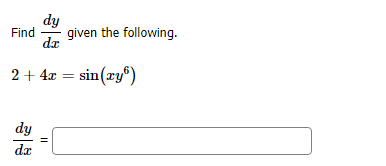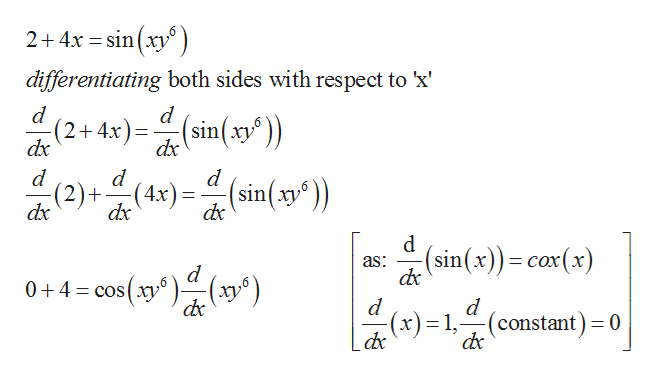Question
1 viewshelp_outlineImage Transcriptionclosedy given the following Find dar 24sin(ry) dy da fullscreen
check_circle

Step 1

The given equation whose derivative is to find is:

Step 2

Now differentiate the equation wit...help_outlineImage Transcriptionclose2+4x sin(xy differentiating both sides with respect to 'x d d (2+4x)(sin(xy) dx dx d d (2)+(4x)(sin(xy)) ху dx dx d (sin(x))cox(x) as: 0 4 cos(xy(y -(x)= 1,-(constant) = 0 fullscreen

### Want to see the full answer?

See Solution

#### Want to see this answer and more?

Solutions are written by subject experts who are available 24/7. Questions are typically answered within 1 hour.*

See Solution
*Response times may vary by subject and question.
Tagged in

### Calculus